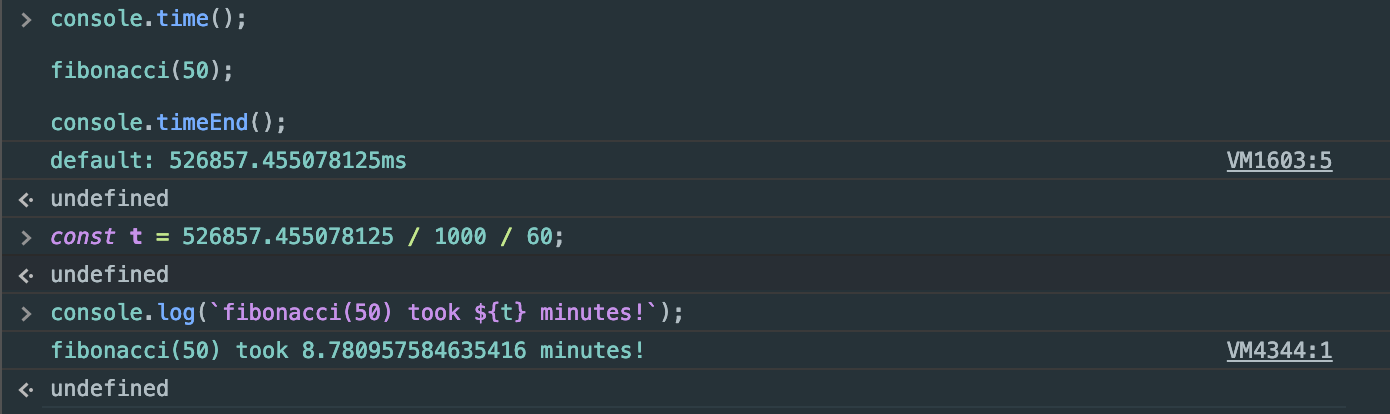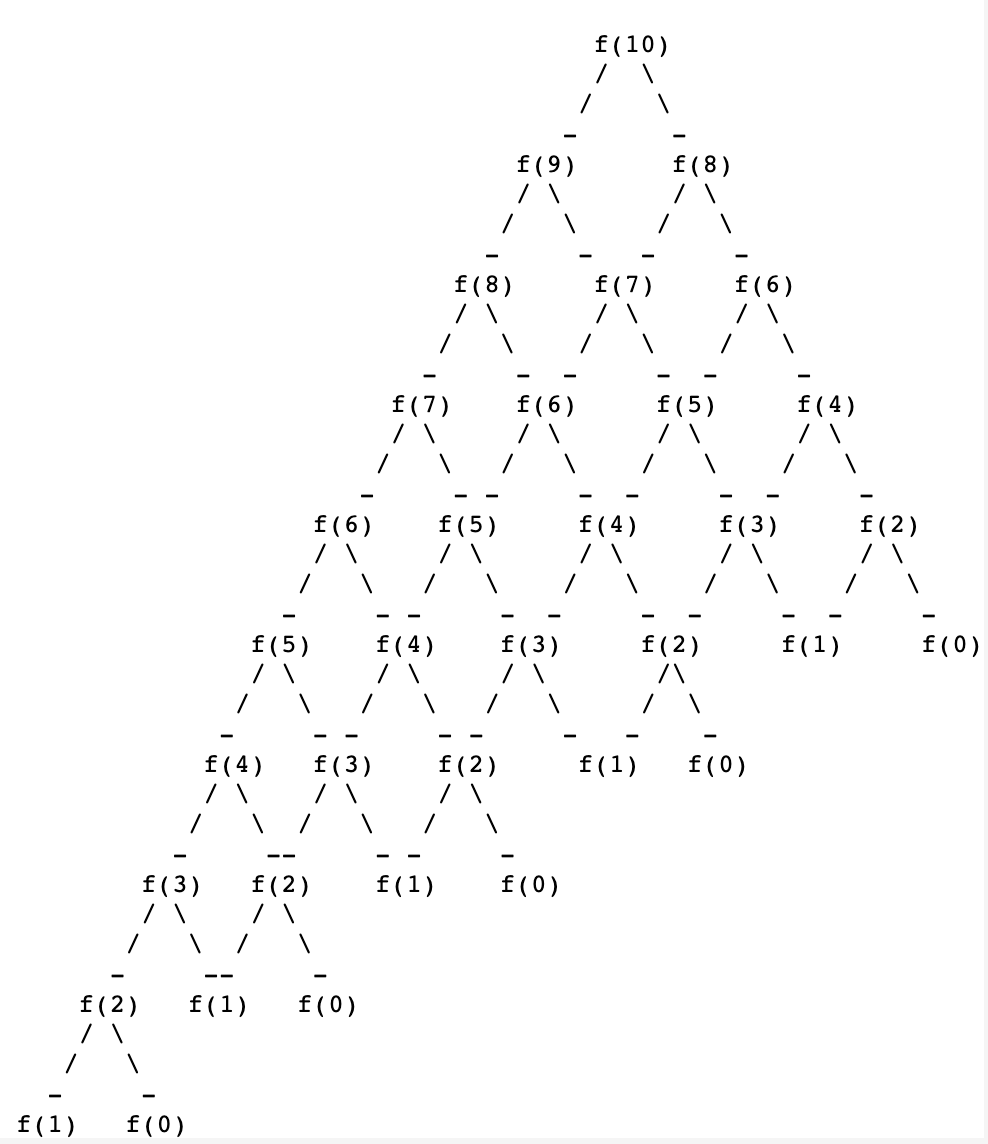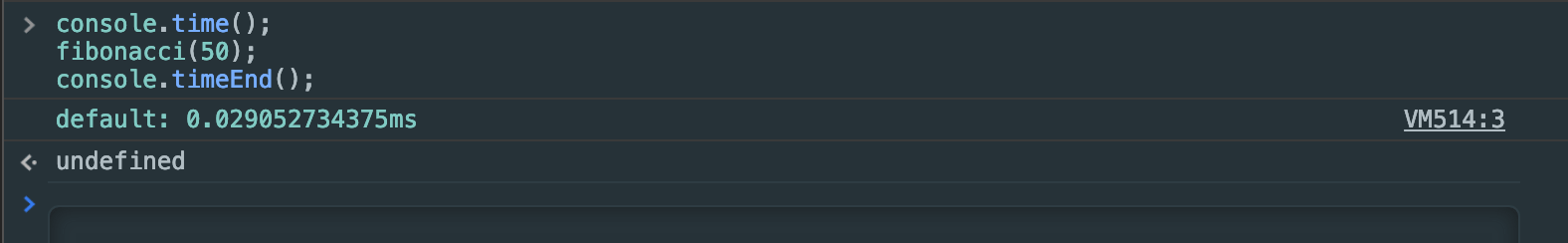# Calculating the nth Fibonacci Number6 min read

A classic interview question: “Please write a function `fibonacci` that takes an integer `n` and returns the nth Fibonacci number.” The Fibonacci sequence follows the following pattern:

``0, 1, 1, 2, 3, 5, 8, 13…``

The pattern continues by adding the previous two Fibonacci numbers together and therefore, the next value above would be `21`. Now let’s write a function to get the `n`th Fibonacci value so that,

``````// base Fibonacci numbers
fibonacci(0) // returns 0
fibonacci(1) // returns 1
// generated Fibonacci numbers
fibonacci(2) // returns 1
fibonacci(3) // returns 2
fibonacci(4) // returns 3
fibonacci(5) // returns 5
fibonacci(6) // returns 8
// ...``````

## Solution 1: Recursion

This is the most popular way of solving this problem because it is easier to reason about since,

``fibonacci(n) = fibonacci(n - 1) + fibonacci(n - 2)``

Let’s write this as a function:

``````function fibonacci(n) {
return fibonacci(n - 1) + fibonacci(n - 2)
}``````

This is great but, this has no stopping condition so it will go on forever. We need to specify that if `n` is `0` or `1` (our base Fibonacci numbers) we return `0` and `1` , respectively.

``````function fibonacci(n) {
if (n === 0) return 0
else if (n === 1) return 1
else return fibonacci(n - 1) + fibonacci(n - 2)
}``````

Great! Try the function out for `n = 1`, `n = 5`, and `n = 50`.

• `fibonacci(1)` should return `1`.
• `fibonacci(5)` should return `5`.
• `fibonacci(50)` should return `12586269025`.

You might have noticed that `fibonacci(50)` hangs in the console for some time. In fact, it took my console around eight minutes to execute!console showing an 8 minute execution of f(50)

This is the downside to this solution. For large `n` , the computation time takes way too long. The second solution fixes this problem.

## Solution 2: Using a Generator Function

So the previous solution worked but, is super slow for large values of `n`. Why is this the case? Well, let’s calculate `fibonacci(10)` as an example by hand (I will denote `fibonacci` as `f` for sake of simplicity.)long recursive tree for f(10)

We are having to dive into a bunch of the same rabbit holes over and over again to calculate `fibonacci(10)`. Why do we have to do this if all we need is the previous two Fibonacci numbers? Is there a way we can just remember the previous two Fibonacci numbers and generate the next Fibonacci number in the sequence? Yes! We can use generators to create an infinite sequence of `Fibonacci` numbers. Generators are interesting. They are like regular functions but with super powers. They are able to return values without completely ending the execution of a function. It does this by making use of the special `yield` statement. Let’s look at a trivial example of a generator function.

``````function* x() {
// the "*" denotes that function x is a generator
yield 'One taught me love'
yield 'One taught me patience'
yield 'And one taught me pain'
}``````

Great! Let’s invoke this function to see how it works:

``````const thanku = x() // we invoke the generator

// invoke the `next` method on the generator prototype
thanku.next() // returns {value: "One taught me love", done: false}
thanku.next() // {value: "One taught me patience", done: false}
thanku.next() // {value: "And one taught me pain", done: false}
thanku.next() // {value: undefined, done: true}

// Now aren't you grateful for your x?``````

For every call to the `next` method on the generator prototype, we get an object with two properties: `value` and `done` which is the value you are yielding from the generator and whether or not your generator is done generating values, respectively. Let’s look at a more interesting example. Let’s generate an infinite sequence of even numbers:

``````function* even() {
let start = 0
yield start // yield 0 as our first even number
while (true) {
// the start of our infinite sequence!
start += 2 // add 2 to start
yield start
}
}

function helper() {
const value = evenNumbers.next()
console.log(`NEXT: \${JSON.stringify(value)}`)
}

const evenNumbers = even()

setTimeout(helper, 1000)
setTimeout(helper, 2000)``````

Let’s go though the execution of the code above together:

1. We first Initialize the variable `evenNumbers` with the invocation of `even` generator.
2. We then wait `1000` milliseconds for the first invocation of `helper`.
3. `1000` milliseconds pass, and `helper` is invoked

1. We initialize `value` with the invocation of `evenNumbers.next`
2. We initialize `start` with `0`
3. Then we `yield` `start` and pause the generator.
4. Now we `console.log` the `value`
4. Wait another `1000` milliseconds for the second invocation of `helper`

1. We enter the `while` loop
2. Increment `start` by 2.
3. `yield` `start` and pause the generator.
4. Now we `console.log` the `value` .

Great! So how do we use the generator function to get the nth Fibonacci number? What we want to do is

1. Create an infinite sequence of Fibonacci numbers using a generator.
2. Keep invoking `Fibonacci.next` until we get the nth Fibonacci number.

### 1. Create an infinite sequence of Fibonacci numbers using a generator

``````function* Fibonacci() {
let a = 0,
b = 1 // base Fibonacci numbers
while (true) {
const c = a + b // next Fibonacci number
yield c
a = b // new a will be what used to be b
b = c // new b will be what used to be c
}
}``````

### 2. Keep invoking `Fibonacci.next` until we have the nth number

We can do this by using a standard `for` loop:

``````function fibonacci(n) {
if (n === 0) return 0
else if (n === 1) return 1
else {
const Fib = Fibonacci()
let value
for (let i = 0; i < n - 1; i++) {
value = Fib.next().value
}
return value
}
}``````

And there you have it: a faster function to find the nth Fibonacci number. Look at the difference in speed! ~8 minutes vs ~0.029 milliseconds!console showing a 0.03 millisecond execution of f(50)### Satoshi

👋 Hello, thanks for the read! If you found my work helpful, have constructive feedback, or just want to say hello, connect with me on social media. Thanks in advance!

© 2021 Made by Scott Iwako